Home
Hostname: page-component-79b67bcb76-6j5sx Total loading time: 0.412 Render date: 2021-05-12T18:53:32.527Z Has data issue: true Feature Flags: { "shouldUseShareProductTool": true, "shouldUseHypothesis": true, "isUnsiloEnabled": true, "metricsAbstractViews": false, "figures": false, "newCiteModal": false, "newCitedByModal": true, "newEcommerce": true }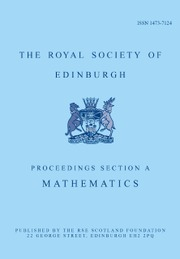Proceedings of the Royal Society of Edinburgh Section A: Mathematics

# Pseudo-differential operators with nonlinear quantizing functions

Published online by Cambridge University Press:  23 January 2019

Corresponding

## Abstract

In this paper we develop the calculus of pseudo-differential operators corresponding to the quantizations of the form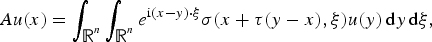$$Au(x)=\int_{{\open R}^n}\int_{{\open R}^n}e^{{\rm i}(x-y)\cdot\xi}\sigma(x+\tau(y-x),\xi)u(y)\,{\rm d}y\,{\rm d}\xi,$$
where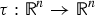$\tau :{\open R}^n\to {\open R}^n$ is a general function. In particular, for the linear choices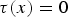$\tau (x)=0$,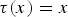$\tau (x)=x$ and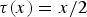$\tau (x)={x}/{2}$ this covers the well-known Kohn–Nirenberg, anti-Kohn–Nirenberg and Weyl quantizations, respectively. Quantizations of such type appear naturally in the analysis on nilpotent Lie groups for polynomial functions τ and here we investigate the corresponding calculus in the model case of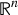${\open R}^n$. We also give examples of nonlinear τ appearing on the polarized and non-polarized Heisenberg groups.

## MSC classification

Type
Research Article
Information
Creative CommonsThis is an Open Access article, distributed under the terms of the Creative Commons Attribution licence (http://creativecommons.org/licenses/by/4.0/), which permits unrestricted re-use, distribution, and reproduction in any medium, provided the original work is properly cited.

## Footnotes

*

Current address: Department of Mathematics: Analysis, Logic and Discrete Mathematics, Ghent University, Belgium and School of Mathematical Sciences, Queen Mary University of London, United Kingdom.

## References

1Bayer, D.. Bilinear time-frequency distributions and pseudodifferential operators. Thesis (Wien: Universität Wien, 2010).Google Scholar
2Calderón, A. and Vaillancourt, R.. On the boundedness of pseudo-differential operators. J. Math. Soc. Japan 23 (1971), 374378.CrossRefGoogle Scholar
3Cappiello, M. and Toft, J.. Pseudo-differential operators in a Gelfand-Shilov setting. Math. Nachr. 290 (2017), 738755.CrossRefGoogle Scholar
4Fischer, V. and Ruzhansky, M.. Quantization on nilpotent Lie groups. Progress in Mathematics, Vol. 314 (Birkhäuser, 2016). (open access book).CrossRefGoogle Scholar
5Lerner, N.. Metrics on the phase space and non-selfadjoint pseudo-differetial operators (Birkhäuser, 2010).CrossRefGoogle Scholar
6Mantoiu, M. and Ruzhansky, M.. Pseudo-differential operators, Wigner transform and Weyl systems on type I locally compact groups. Doc. Math. 22 (2017), 15391592.Google Scholar
7Ruzhansky, M. and Sugimoto, M.. On global inversion of homogeneous maps. Bull. Math. Sci. 5 (2015), 1318.CrossRefGoogle Scholar
8Ruzhansky, M. and Sugimoto, M.. Global L 2-boundedness theorems for a class of Fourier integral operators. Comm. Partial Diff. Equ. 31 (2006), 547569.CrossRefGoogle Scholar
9Ruzhansky, M. and Turunen, V.. Pseudo-differential operators and symmetries (Birkhäuser, 2010).CrossRefGoogle Scholar
10Shubin, M. A.. Pseudodifferential operators and spectral theory (Springer, 2001).CrossRefGoogle Scholar
11Stein, E. M.. Harmonic analysis: real-variable methods, orthogonality, and oscillatory integrals (Princeton: Princeton University Press, 1993).Google Scholar
12Taylor, M. E.. Partial differential equations II. Qualitative studies of linear equations, 2nd edn. Applied Mathematical Sciences, 116 (New York: Springer, 2011).Google Scholar
13Toft, J.. Matrix parameterized pseudo-differential calculi on modulation spaces. Generalized functions and Fourier analysis, 215–235, Oper. Theory Adv. Appl., 260, Adv. Partial Differ. Equ. (Basel) (Cham: Birkhäuser/Springer, 2017).CrossRefGoogle ScholarYou have AccessOpen access

# Send article to Kindle

Note you can select to send to either the @free.kindle.com or @kindle.com variations. ‘@free.kindle.com’ emails are free but can only be sent to your device when it is connected to wi-fi. ‘@kindle.com’ emails can be delivered even when you are not connected to wi-fi, but note that service fees apply.

Find out more about the Kindle Personal Document Service.

Pseudo-differential operators with nonlinear quantizing functions
Available formats
×

# Send article to Dropbox

To send this article to your Dropbox account, please select one or more formats and confirm that you agree to abide by our usage policies. If this is the first time you use this feature, you will be asked to authorise Cambridge Core to connect with your <service> account. Find out more about sending content to Dropbox.

Pseudo-differential operators with nonlinear quantizing functions
Available formats
×

# Send article to Google Drive

To send this article to your Google Drive account, please select one or more formats and confirm that you agree to abide by our usage policies. If this is the first time you use this feature, you will be asked to authorise Cambridge Core to connect with your <service> account. Find out more about sending content to Google Drive.

Pseudo-differential operators with nonlinear quantizing functions
Available formats
×
×